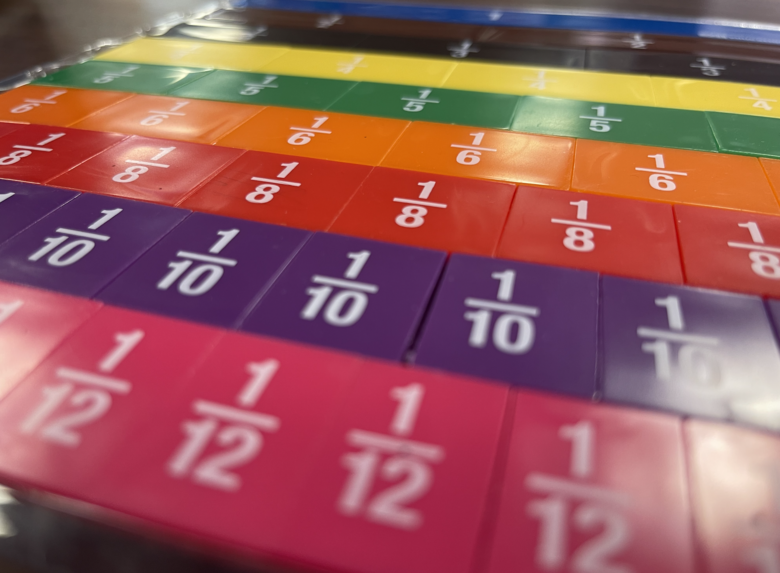Author:
Josalyn
Subject:
Elementary Mathematics
Material Type:
Activity/Lab
Level:
Lower Elementary
Tags:
Lesson Plan
Language:
English
Media Formats:
Text/HTML

# Finding Equivalent Fractions## Overview

In this lesson students will practice creating equivalent fractions. Using manipulatives and can be done with pencil and paper. This is a face to face lesson.

The program that was used for support is IReady - Grade 3 Instruction

Assessment - There is an observational assessment, and then a virtual practice from boom learning

The image on the cover is a picture of my fraction tiles in my classroom.

# Purpose

In this lesson students solve a problem that requires showing that two fractions are equivalent. Students model and compare fractions. Students use these models to show how two fractions are equivalent.

This lesson will take 35 minutes and is meant for face to face instruction.

# Teacher Notes

This lesson will reinforce the concept that two different fractions can name the same part of a whole. During this lesson you will need fraction tiles both round, and rectangle, you may also like graphing paper. Students will have misconceptions about the fractions they can compare. It is important they first compare using a model with the same size and kind. Such as number line to number line. Review part, whole, numerator, denominator, model, number line.

# Objectives and Success CriteriaObjective: I can identify two fractions that are equivalent using a model or number line.

Success Criteria: I will know that I am able to do this when I can identify fractions that are equivalent using a number line and model for evidence of equivalency

# Background KnowledgeReview the parts of a fraction, using a rectangle with 4 parts. Then add a line down the middle that will seperate the fraction into 8 parts. Discuss the different fractions that are availabe in the model with 4 parts and how they compare to the fraction when the model has 8 parts.

# InstructionPose the question

Carl eats 2/8 of an orange. Trey's orange is the same size, and he eats 1/4 of it. Show that the two boys eat the same amount of orange.

During this time let students try, and draw

Come together and have them share what they chose. Choose a student who has done it but is almost there, then have a student that has the answer as you are looking for. Then relate the two.

Partner 2: I chose to _______ because ______ it helped me to ______

Review question showing students how to compare using a number line and fraction tiles

As you review, continue to express equal sizes.

Practice with the students

Pass out tiles and graphing paper. Give them sets of fractions to create equivalent fractions

3/4 = 6/8

2/3 = 4/6

1/3 = 2/6

2/3 = 4/6

# AssessmentSmall group assessment - Pull small groups of students give a fraction have students build them and then build and edquivalent fraction

1/2 = 4/8

1/4 = 2/8

6/8 = 3/4